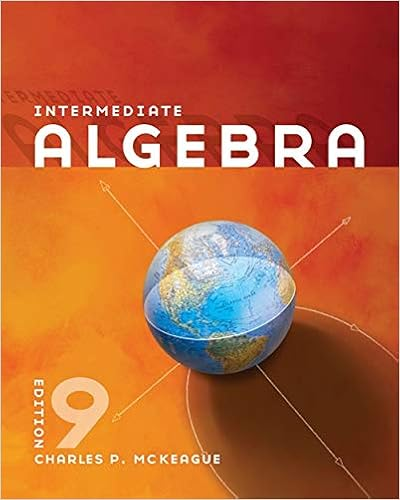# Week+2-Chapter+2+In+class+Activities+A+(1)_Vargovich.docx -...

• Homework Help
• 3
• 100% (4) 4 out of 4 people found this document helpful

This preview shows page 1 - 3 out of 3 pages.

##### We have textbook solutions for you!
The document you are viewing contains questions related to this textbook.The document you are viewing contains questions related to this textbook.
Chapter 11 / Exercise 6
Intermediate Algebra
MckeagueExpert Verified
Math 040: Re-Entry Mathematics Franklin UniversityWeek 2 Chapter 2 In class ActivitiesName: ___Chandra Vargovich______ 1.Fill in the blank with < or > to make a true statementa.- 8 > - 9 b.- 226 < 1982.Find the oppositea.31 __-31_________b.-612 _612__________3.Simplify the expressiona.– (-15) _15________b.|79|_-79____c.– (34) __34_______d.|47|____-47_____4.Fill in the blank with <, >, or =a.– ( – 4) _>____ |4|b.– 44 ___>____ – 54c.|55|___<___ – ( – 65) 5.Add the numbers a.23 + 12 ___35_______b.78 + (-33) ___45______c.-105 + ( -23) __-128______d.-475 + (623) __148______6.Simplifya.20 + (−12) + (−5) ___3___________b.−23 + (−4) + (−12) + (−5) _____-44_______c.4 + (−12) + (−30) + 16 + 10 ___-12__________7.A contestant on Jeopardy scored the following amounts for several questions he answered. \$200, – \$400, \$1000, – \$400, \$600 Determine his total score._(-\$200)+(-\$400)+\$1000+(-\$400)+\$600=\$600________________________________________ 8.Simplifya.35 (– 56) = __91_________b.–320 – (–198) = _-122__________c.–40 + 605 – 815 = __-250________d.–21 – 19 + (– 27) + 45 – (– 16) = ____-16__________ 9.Temperatures on the Moon range from −184°C during its night to 214°C during its day. Find the difference between the highest temperature on the Moon and the lowest temperature. _214°C - (-184°C) = 398°C _____________________ 1030315
##### We have textbook solutions for you!
The document you are viewing contains questions related to this textbook.The document you are viewing contains questions related to this textbook.
Chapter 11 / Exercise 6
Intermediate Algebra
MckeagueExpert Verified
Math 040: Re-Entry Mathematics Franklin University10. Multiply the integers a.– 17( –8) = ___136_______________b.– 6(23) = _____-136______________c.–41 0 = ____0________________d.67 (–2) = __-134_________________ 11. Multiply a.(–11)(–5)(–3) = __-165_______________________b.(–8)(–4)(0)( –2) = __0_____________________12. Simplify a.
•••